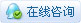##帐号 自动登录 找回密码 密码 论坛注册
 搜索

# [分享] 简单地将IP地址转换为十六进制发表于 2019-9-10 12:01:36 | 显示全部楼层 |阅读模式本帖最后由 thegreek1 于 2019-9-10 12:03 编辑 记住这张桌子 0 = 0 1 = 1 2 = 2 3 = 3 4 = 4 5 = 5 6 = 6 7 = 7 8 = 8 9 = 9 10 = A. 11 = B. 12 = C. 13 = D. 14 = E. 15 = F. 192 = C0 = (192/16) = 12.0 = take the remainder (0 x 16) = 0 to convert it to hexadecimal (0) Then take the result (12) divided by 16 (12/16), if it is less than 1, then Convert the remainder to hex 12 = C and then add it back to C0 168 = A8 = (168/16) = 10.8 = remainder (.8 x 16) = 12.8 converts it to hexadecimal (A) and then Take the result (12) divided by 16 (12/16), if it is less than 1, then just cover The remainder is hexadecimal 0 = 0 and then add 0A8 or A8 backwards 42 = 2A = (42/16) = 2.625 = remainder (.625 x 16) = 10 converts it to hexadecimal (A) and then Take the result (2) divided by 16 (2/16), if it is less than 1, then just hide it The remainder is hexadecimal 2 = 2 and then added back to 2A发表于 2019-9-10 16:55:18 | 显示全部楼层
 Thanks for sharing  !!!发表于 2019-10-12 11:08:51 | 显示全部楼层
 666666666666

 本版积分规则 回帖后跳转到最后一页|Archiver|手机版|小黑屋|sitemap|鸿鹄论坛 ( 京ICP备14027439号 )

GMT+8, 2020-1-20 12:20 , Processed in 0.073044 second(s), 7 queries , Gzip On, MemCache On.